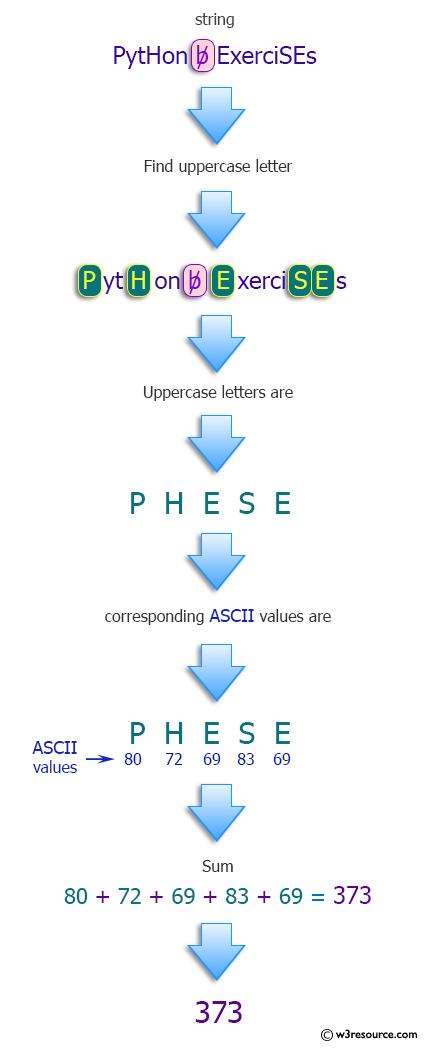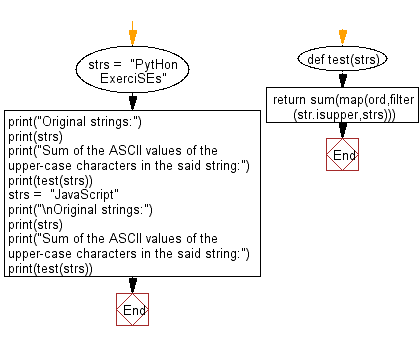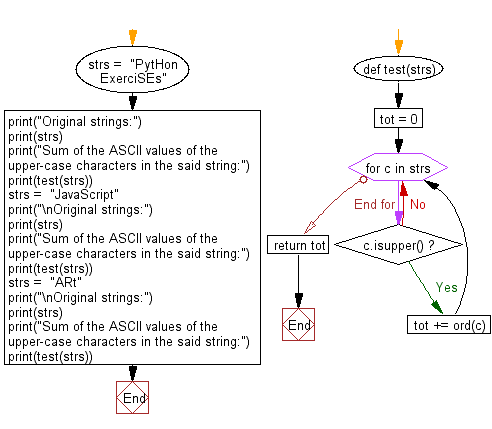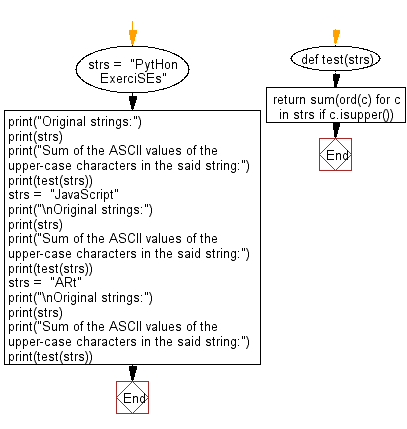﻿ Python: Compute the sum of the ASCII values of the upper-case characters in a given string - w3resource# Python: Compute the sum of the ASCII values of the upper-case characters in a given string

## Python Programming Puzzles: Exercise-22 with Solution

Write a Python program to compute the sum of the ASCII values of the upper-case characters in a given string.

```Input:
PytHon ExerciSEs
Output:
373

Input:
JavaScript
Output:
157
```

Pictorial Presentation:Sample Solution-1:

Python Code:

``````#License: https://bit.ly/3oLErEI

def test(strs):
return sum(map(ord,filter(str.isupper,strs)))
strs =  "PytHon ExerciSEs"
print("Original strings:")
print(strs)
print("Sum of the ASCII values of the upper-case characters in the said string:")
print(test(strs))
strs =  "JavaScript"
print("\nOriginal strings:")
print(strs)
print("Sum of the ASCII values of the upper-case characters in the said string:")
print(test(strs))

``````

Sample Output:

```Original strings:
PytHon ExerciSEs
Sum of the ASCII values of the upper-case characters in the said string:
373

Original strings:
JavaScript
Sum of the ASCII values of the upper-case characters in the said string:
157
```

Flowchart:## Visualize Python code execution:

The following tool visualize what the computer is doing step-by-step as it executes the said program:

Sample Solution-2:

Python Code:

``````#License: https://bit.ly/3oLErEI
def test(strs):
tot = 0
for c in strs:
if c.isupper():
tot += ord(c)
strs =  "PytHon ExerciSEs"
print("Original strings:")
print(strs)
print("Sum of the ASCII values of the upper-case characters in the said string:")
print(test(strs))
strs =  "JavaScript"
print("\nOriginal strings:")
print(strs)
print("Sum of the ASCII values of the upper-case characters in the said string:")
print(test(strs))
strs =  "ARt"
print("\nOriginal strings:")
print(strs)
print("Sum of the ASCII values of the upper-case characters in the said string:")
print(test(strs))
``````

Sample Output:

```Original strings:
PytHon ExerciSEs
Sum of the ASCII values of the upper-case characters in the said string:
373

Original strings:
JavaScript
Sum of the ASCII values of the upper-case characters in the said string:
157

Original strings:
ARt
Sum of the ASCII values of the upper-case characters in the said string:
147
```

Flowchart:## Visualize Python code execution:

The following tool visualize what the computer is doing step-by-step as it executes the said program:

Sample Solution-3:

Python Code:

``````#License: https://bit.ly/3oLErEI
def test(strs):
return sum(ord(c) for c in strs if c.isupper())

strs =  "PytHon ExerciSEs"
print("Original strings:")
print(strs)
print("Sum of the ASCII values of the upper-case characters in the said string:")
print(test(strs))
strs =  "JavaScript"
print("\nOriginal strings:")
print(strs)
print("Sum of the ASCII values of the upper-case characters in the said string:")
print(test(strs))
strs =  "ARt"
print("\nOriginal strings:")
print(strs)
print("Sum of the ASCII values of the upper-case characters in the said string:")
print(test(strs))
``````

Sample Output:

```Original strings:
PytHon ExerciSEs
Sum of the ASCII values of the upper-case characters in the said string:
373

Original strings:
JavaScript
Sum of the ASCII values of the upper-case characters in the said string:
157

Original strings:
ARt
Sum of the ASCII values of the upper-case characters in the said string:
147
```

Flowchart:## Visualize Python code execution:

The following tool visualize what the computer is doing step-by-step as it executes the said program:

Python Code Editor :

Have another way to solve this solution? Contribute your code (and comments) through Disqus.

What is the difficulty level of this exercise?

Test your Programming skills with w3resource's quiz.

﻿

## Python: Tips of the Day

Clamps num within the inclusive range specified by the boundary values x and y:

Example:

```def tips_clamp_num(num,x,y):
return max(min(num, max(x, y)), min(x, y))
print(tips_clamp_num(2, 4, 6))
print(tips_clamp_num(1, -1, -6))
```

Output:

```4
-1
```